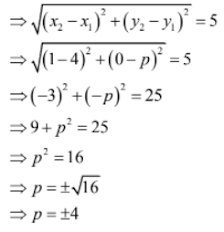# RS Aggarwal Solutions Chapter 16 Coordinate Geometry MCQ Class 10 Maths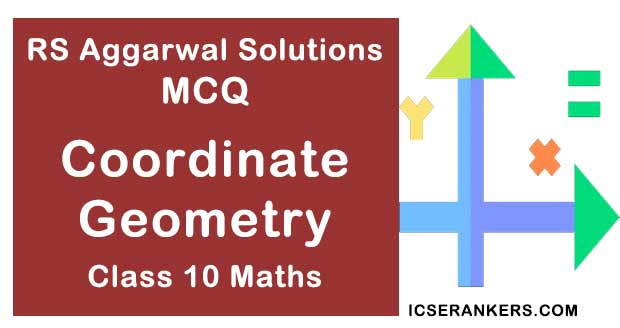Chapter Name RS Aggarwal Chapter 16 Coordinate Geometry Book Name RS Aggarwal Mathematics for Class 10 Other Exercises Exercise 16AExercise 16BExercise 16CExercise 16D Related Study NCERT Solutions for Class 10 Maths

### Coordinate Geometry MCQ Solutions

1. The distance of the point P(-6, 8) from the origin is

(a) 8

(b) 2√ 7

(c) 6

(d) 10

Solution

(d) 10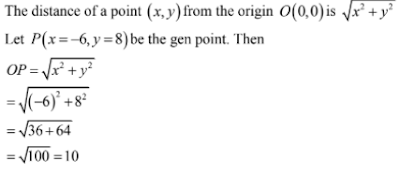2. The distance of the point (-3, 4) from the x-axis is

(a) 3

(b) -3

(c) 4

(d) 5

Solution

The distance of a point (x, y) from x-axis is |y|.

Here, the point is (-3, 4). So, its distance from x-axis is |4| = 4

3. The point on x-axis which is equidistant from the points A(-1, 0) and B(5, 0) is

(a) (0, 2)

(b) (2, 0)

(c) (3, 0)

(d) (0, 3)

Solution

(b) (2, 0)

Let P(x, 0) the point on x-axis, then

AP = BP ⇒ AP2 = BP2

⇒ (x + 1)2 + (0 + 0)2 = (x – 5)2 + (0 – 0)2

⇒ x+ 2x + 1 = x2 – 10x + 25

⇒ 12x = 24

⇒ x = 2

Thus, the required point is (2, 0).

4. If R(5, 6) is the midpoint of the line segment AB joining the points A(6, 5) and B(4, 4) then y equals

(a) 5

(b) 7

(c) 12

(d) 6

Solution

(b) 7

Since R(5, 6) is the midpoint of the line segment AB joining the points

A(6, 5) and B(4, y), therefore,

(5 + y)/2 = 6

⇒ 5 + y = 12

⇒ y = 12 – 5 = 7

5. If the point C(k, 4) divides the join of the points A(2, 6) and B(5, 1) in the ratio 2 : 3 then the value of k is

(a) 16

(b) 28/5

(c) 16/5

(d) 8/5

Solution

(c) 16/5

The point C(k, 4) divides the join of the points A(2, 6) and B(5, 1) in the ratio 2 : 3. So,

k = (2 × 5 + 3 × 2)/(2 + 3) = (10 + 6)/5

= 16/5

6. The perimeter of the triangle with vertices (0, 4), (0, 0) and (3, 0) is

(a) (7 + √5)

(b) 5

(c) 10

(d) 12

Solution

(d) 12

Let A(0, 4), B(0, 0) and C(3, 0) be the given vertices. So,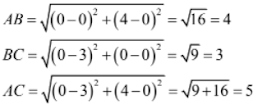Therefore,

AB + BC + AC = 4 + 3 + 5 = 12.

7. If A(1, 3), B(-1, 2), C(2, 5) and D(x, 4) are the vertices of a ||gm ABCD the value of x is

(a) 3

(b) 4

(c) 0

(d) 3/2

Solution

(b) 4

The diagonals of a parallelogram bisect each other. The vertices of the ||gm ABCD are A(1, 3), B(-1, 2) and C(2, 5) and D(x, 4)

Here, AC and BD are the diagonals. So

(1 + 2)/2 = (-1 + x)/2

⇒ x – 1 = 3

⇒ x = 1+ 3 = 4

8. If the points A(x, 2), B(-3, -4) and C(7, -5) are collinear then the value of x is

(a) -63

(b) 63

(c) 60

(d) – 60

Solution

(a) – 63

Let A(x1 = x, y= 2), B(x2 = -3, y= -4) and C(x= 7, y3 = -5) be collinear points. Then

x1(y2 – y3) + x2(y3 – y1) + x3(y– y2) = 0

⇒ x( - 4 + 5) + (-3) (-5 – 2) + 7(2 + 4) = 0

⇒ x + 21 + 42 = 0

⇒ x = -63

9. The area of a triangle with vertices A(5, 0), B(8, 0) and C(8, 4) in square units is

(a) 20

(b) 12

(c) 6

(d) 16

Solution

(c) 6

Let A (x= 5, y1 = 0), B(x= 8, y= 0) and C(x3 = 8, y3 = 4) be the vertices of the triangle.

Then

Area(△ABC) = ½[x1(y2 – y3) + x2(y3 – y1) + x3(y1 – y2)]

= ½[5(0 – 4) + 8(4 – 0) + 8(0 – 0)]

= ½[-20 + 32 + 0]

= 6 sq. units.

10. The area of ABC with vertices A(a, 0), O(0, 0) and B(0, b) in square units is

(a) ab

(b) ½.ab

(c) ½.a2b2

(d) 1/2b2

Solution

(b) 1/2ab

Let A(x= a, y1 = 0), O(x= 0, y2 = 0) and B(x3 = 0, y3 = b) be the given vertices. So

Area (△ABO) = ½|x1(y2 – y3) + x2(y3 – y1) + x3(y1 – y2)|

= ½|a(0 – b) + 0(b – 0) + 0(0 – 0)|

= ½|-ab|

= 1/2ab

11. If P(a/2, 4) is the midpoint of the line segment joining the points A(-6, 5) and B(-2, 3) then the value of a is

(a) -8

(b) 3

(c) -4

(d) 4

Solution

(a) - 8

The point P(a/2, 4) is the midpoint of the line segment joining the points A(-6, 5) and B(-2, 3).

So, a/2 = (-6 – 2)/2

⇒ a/2 = -4

⇒ a = -8

12. ABCD is a rectangle whose three vertices are B(4, 0), C(4, 3) and D(0, 3). The length of one of its diagonals is

(a) 5

(b) 4

(c) 3

(d) 245

Solution

(a) 5

Here, AC and BD are two diagonals of the rectangle ABCD. So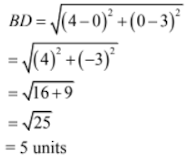13. The coordinates of the point P dividing the line segment joining the points A(1, 3) and B(4, 6) in the ratio 2 : 1 is

(a) (2, 4)

(b) (3, 5)

(c) (4, 2)

(d) (5, 3)

Solution

(b) (3, 5)

Here, the point P divides the line segment joining the points A(1, 3) and B(4, 6) in the ratio 2 : 1. Then,

Coordinates of P = (2 × 4 + 1 × 1)/(2 + 1), (2 × 6 + 1 × 3)/(2 + 1)

= (8 + 1)/3, (12 + 3)/3

= (9/3, 15/3)

= (3, 5)

14. If the coordinates of one end of a diameter of a circle are (2, 3) and the coordinates of its centre are (-2, 5), then the coordinates of the other end of the diameter are

(a) (-6, 7)

(b) (6, -7)

(c) (4, 2)

(d) (5, 3)

Solution

(a) (-6, 7)

Let (x, y) be the coordinates of the other end of the diameter. Then

-2 = (2 + x)/2

⇒ x = -6

5 = (3 + y)2

⇒ y = 7

15. In the given figure P(5, -3) and Q(3, y) are the points of trisection of the line segment joining A(7, -2) and B(1, -5). Then, y equals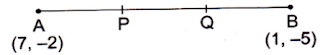(a) 2

(b) 4

(c) -4

(b) -5/2

Solution

(c) -4

Here, AQ : BQ = 2 : 1. Then,

y = {2 × (-5) + 1 × (-2)}/(2 + 1)

= (-10 – 2)/3

= -4

16. The midpoint of segment AB is P(0, 4). If the coordinates of B are(-2, 3), then the coordinates of A are

(a) (2, 5)

(b) (-2, -5)

(c) (2, 9)

(d) (-2, 11)

Solution

(a) (2, 5)

Let (x, y) be the coordinates of A. Then,

0 = (-2 + x)/2

⇒ x = 2

4 = (3 + y)/2

⇒ y = 8 – 3 = 5

Thus, the coordinates of A are (2, 5).

17. The point P which divides the line segment joining the points A(2, -5) and B(5, 2) in the ratio 2 : 3 lies in the quadrant

(a) I

(b) II

(c) III

(d) IV

Solution

(d) IV

Let (x, y) be the coordinates of P. Then,

x = (2 × 5 + 3 × 2)/(2 + 3) = (10 + 6)/5 = 16/5

y = (2 × 2 + 3 × (-5)/(2 + 3) = (4 – 15)/5 = -11/5

Thus, the coordinates of point P are (16/5, -11/5) and so it lies in the fourth quadrant.

18. If A(-6, 7) and B(-1, -5) are two given points then the distance 2AB is

(a) 13

(b) 26

(c) 169

(d) 238

Solution

(b) 26

The given points are A(-6, 7) and B(-1, -5). So,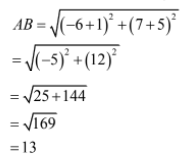Thus, 2AB = 26.

19. Which point on x-axis is equidistant from the points A(7, 6) and B(-3, 4)

(a) (0, 4)

(b) (-4, 0)

(c) (3, 0)

(d) (0, 3)

Solution

(c) (3, 0)

Let p(x, 0) be the point on x-axis. Then as per the question

AP = BP ⇒ AP2 = BP2

⇒ (x – 7)2 + (0 – 6)2 = (x – 3)+ (0 – 4)2

⇒ x2 – 14x + 49 + 36 = x+ 6x + 9 + 16

⇒ 60 = 20x

⇒ x = 60/20 = 3

Thus, the required point is (3, 0).

20. The distance of P(3, 4) from the x-axis is

(a) 3 units

(b) 4 units

(c) 5 units

(d) 1 unit

Solution

(b) 4 units

The y-coordinate the distance of the point from the x-axis

Here, the y-coordinate is 4.

21. In what ratio does the x-axis divide the join of A(2, -3) and B(5, 6) ?

(a) 2 : 3

(b) 3 : 5

(c) 1 : 2

(d) 2 : 1

Solution

(c) 1 : 2

Let AB be divided by the x-axis in the ratio k : 1 at the point P.

Then, by section formula, the coordinates of P are

P(5k + 2)/(k + 1), (6k – 3)/(k + 1)

But P lies on the x-axes so, its ordinate is 0.

(6k – 3)/(k + 1) = 0

⇒ 6k – 3 = 0

⇒ 6k = 3

⇒ k = 1/2

Hence, the required ratio is 1/2 : 1 which is same as 1 : 2.

22. In what ratio does the y-axis divide the join of P(-4, 2) and Q(8, 3) ?

(a) 3 : 1

(b) 1 : 3

(c) 2 : 1

(d) 1 : 2

Solution

(d) 1 : 2

Let AB be divided by the y-axis in the ratio k : 1 at the point P.

Then, by section formula, the coordinates of P are

P(8k – 4)/(k + 1), (3k + 2)/(k + 1)

But, P lies on the y-axis, so, its abscissa is 0.

⇒ (8k – 4)/(k + 1) = 0

⇒ 8k – 4 = 0

⇒ 8k = 4

⇒ k = 1/2

Hence, the required ratio is ½: 1 which is same as 1 : 2.

23. If P(-1, 1) is the midpoint of the line segment joining A(-3, b) and B(1, b +4) then b = ?

(a) 1

(b) -1

(c) 2

(d) 0

Solution

(b) -1

The given points are A(-3, b) and B(1, b + 4)

Then, (x= -3, y1 = b) and (x2 = 1, y2 = b + 4)

Therefore,

x = [-3 + 1]/2

= -2/2

= - 1

And

y = [b + (b + 4)]/2

= (2b + 4)/2

= b + 2

But the midpoint is P(-1, 1).

Therefore,

b + 2 = 1

⇒ b = -1

24. The line 2x + y – 4 = 0 divide the line segment joining A(2, -2) and B(3, 7) in the ratio

(a) 2 : 5

(b) 2 : 9

(c) 2 : 7

(d) 2 : 3

Solution

(b) 2 : 9

Let the line 2x + y – 4 = 0 divide the line segment in the ratio k : 1 at the point P.

Then, by section formula the coordinates of P are

P(3k + 2)/(k + 1), (7k – 2)/(k + 1)

Since P lies on the line 2x + y – 4 = 0, we have

2(3k + 2)/(k + 1) + (7k – 2)/(k + 1) – 4 = 0

⇒ (6k + 4) + (7k – 2) – (4k + 4) = 0

⇒ 9k = 2

⇒ k = 2/9

Hence, the required ratio is 2/9 : 1 which is same as 2 : 9.

25. If A(4, 2), B(6, 5) and C(1, 4) be the vertices of ABC and AD is a median, then the coordinates of D are

(a) (5/2, 3)

(b) (5, 7/2)

(c) (7/2, 9/2)

(d) none of these

Solution

(c) (7/2, 9/2)

D is the midpoint of BC

So, the coordinates of D are

D(6 + 1)/2, (5 + 4) [B(6, 5) and C(1, 4)

⇒ (x1 = 6, y1 = 5) and (x2 = 1, y= 4)]

i.e., D(7/2, 9/2)

26. If A(-1, 0), B(5, -2) and C(8, 2) are the vertices of ABC then its centroid is

(a) (12, 0)

(b) (6, 0)

(c) (0, 6)

(d) (4, 0)

Solution

(d) (4, 0)

The given point are A(-1, 0), B(5, -2) and C(8, 2).

Here, (x1 = -1, y = 0), (x2 = 5, y = -2) and (x3 = 8, y3 = 2)

Let G(x, y) be the centroid of △ABC. Then,

x = 1/3(x1 + x+ x3)

= 1/3(-1 + 5 + 8)

= 4

and

y = 1/3(y1 + y2 + y3)

= 1/3(0 – 2 + 2)

= 0

Hence, the centroid of △ABC is G(4, 0).

27. Two vertices of ABC are A(-1, 4) and B(5, 2) and its centroid is G(0, -3). Then the coordinates of C are

(a) (4, 3)

(b) (4, 15)

(c) (-4, 15)

(d) (-15, -4)

Solution

(c) (-4, -15)

Two vertices of △ABC are A(-1, 4) and B(5, 2).

Let the third vertex be C(a, b).

Then, the coordinates of its centroid are

G(-1 + 5 + a)/3, (4 + 2 + b)/3

i.e., G(4 + a)/3, (6 + b)/3

But it is given that the centroid is G(0, -3)

Therefore,

(4 + a)/3 = 0 and (6 + b)/3 = - 3

⇒ 4 + a = 0 and 6 + b = -9

⇒ a = -4 and b = 15

Hence, the third vertex of △ABC is C(-4, -15)

28. The points A(-4, 0), B(4, 0) and C(0, 3) are the vertices of a triangle, which is

(a) isosceles

(b) equilateral

(c) scalene

(d) right-angled

Solution

(a) isosceles

Let A(-4, 0), B(4, 0) and C(0, 3) be the given points. Then,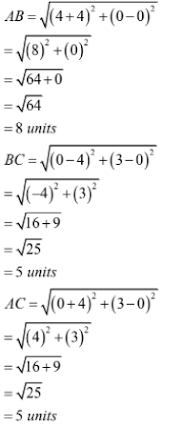BC = AC = 5 units

Therefore, △ABC is isosceles

29. The points P(0, 6), Q(-5, 3) and R(3, 1) are the vertices of a triangle, which is

(a) equilateral

(b) isosceles

(c) scalene

(d) right-angled

Solution

(d) right-angled

Let P(0, 6), Q(-5, 3) and R(3, 1) be the given points. Then,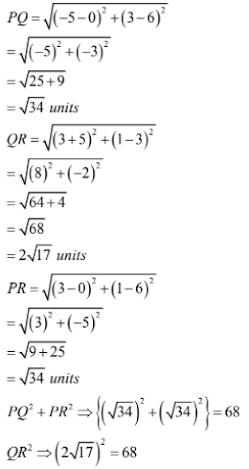Thus, PQ2 + PR2 = QR2

Therefore, △PQR is right-angled.

30. If the points A(2, 3), B(5, k) and C(6, 7) are collinear then

(a) k = 4

(b) k = 6

(c) k = -3/2

(d) k = 11/4

Solution

(b) k = 6

The given points are A(2, 3),B(5, k) and C(6, 7)

Here, (x1 = 2, y1 = 3), (x= 5, y= k) and (x3 = 6, y3 = 7).

Point A, B and C are collinear. Then,

x1(y– y3) + x2(y3 – y1) + x3(y1 – y2) = 0

⇒ 2(k – 7) + 5(7 – 3) + 6(3 – k) = 0

⇒ 2k – 14 + 20 + 18 – 6k = 0

⇒ - 4k = -24

⇒ k = 6

31. If the point A(1, 2), O(0, 0) and C(a, b) are collinear, then

(a) a = b

(b) a = 2b

(c) 2a = b

(d) a + b = 0

Solution

(c) 2a = b

The given points are A(1, 2), O(0, 0) and C(a, b)

Here, (x= 1, y1 = 2), (x2 = 0, y= 0) and (x3 = a, y= b)

Point A, O and C are collinear

⇒ x1(y2 – y3) + x2(y– y1) + x3(y1 – y2) = 0

⇒ 1(0 – b) + 0(b – 2|) + a(2 – 0) = 0

⇒ - b + 2a = 0

⇒ 2a = b

32. The area of ABC with vertices A(3, 0), B(7, 0) and C(8, 4) is

(a) 14 sq units

(b) 28 sq units

(c) 8 sq units

(d) 6 sq units

Solution

(c) 8 sq units

The given points are A(3, 0), B(7, 0) and C(8, 4).

Here, (x= 3, y1 = 0), (x2 = 7, y2 = 0) and (x3 = 8, y3 = 4)

Therefore,

Area of △ABC = ½[x1(y– y3) + x2(y– y1) + x3(y1 – y2)]

= ½[3(0 – 4) + 7(4 – 0) + 8(0 – 0)]

= ½[-12 + 28 + 0]

= (1/2 × 16)

= 8 sq. units

33. AOBC is rectangle whose three vertices A(0, 3), O(0, 0) and B(5, 0). The length of each of its diagonals is

(a) 5 units

(b) 3 units

(c) 4 units

(d) √34 units
Solution

(c) 4 units

A(0, 3), O(0, 0) and B(5, 0) are the three vertices of a rectangle. Let C be the fourth vertex

Then, the length of the diagonal,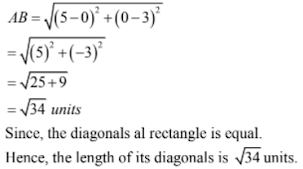34.
If the distance between the points A(4, p) and B(1, 0) is 5 then

(a) p = 4 only

(b) p = - 4

(c) p = ± 4

(d) p = 0

Solution

(c) p = ± 4

The given points are A(4, p) and B(1, 0) and AB = 5

Then, (x1 = 4, y1 = p) and (x= 1, y2 = 0)

Therefore,

AB = 5